# Expressões¶

The Expressions feature is available from many parts in QGIS. It can be accessed using the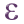Expression Builder, the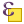Select By Expression..., the Geometry generator symbol layer option, the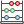Field calculator or the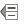Data defined override tool. Based on layer data and prebuilt or user defined functions, it offers a powerful way to manipulate attribute value, geometry and variables in order to dynamically change the geometry style, the content or position of the label, the value for diagram, the height of a composer item, select some features, create virtual field ...

Alguns exemplos:

• From Field Calculator, calculate a “pop_density” field using existing “total_pop” and “area_km2” fields:

```"total_pop" / "area_km2"
```
• Update the field “density_level” with categories according to the “pop_density” values:

```CASE WHEN "pop_density" < 50 THEN 'Low population density'
WHEN "pop_density" >= 50 and "pop_density" < 150 THEN 'Medium population density'
WHEN "pop_density" >= 150 THEN 'High population density'
END```
• Apply a categorized style to all the features according to whether their average house price is smaller or higher than 10000€ per square metre:

```"price_m2" > 10000
```
• Using the “Select By Expression...” tool, select all the features representing areas of “High population density” and whose average house price is higher than 10000€ per square metre:

```"density_level" = 'High population density' and "price_m2" > 10000
```

Likewise, the previous expression could also be used to define which features should be labeled or shown in the map. Using expressions offers you a lot of possibilities.

• Expression tab which lists functions to use
• Function Editor tab which helps to create custom functions to use in the expressions.

## Lista de funções¶

Figure Expression 1: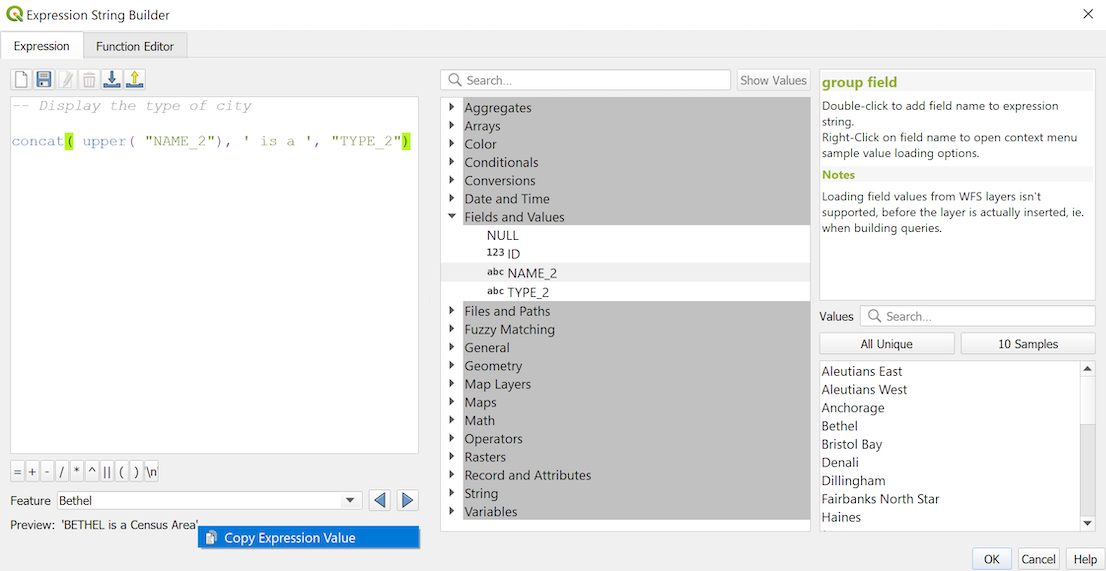The Expression tab

The Expression tab contains functions as well as layer’s fields and values. It contains widgets to:

• type expressions using functions and/or fields. A preview of the expression’s result is displayed at the bottom of the dialog.
• select the appropriate function among a list. A search box is available to filter the list and quickly find a particular function or field. Double-clicking on the item’s name adds it to the expression being written.
• display help for each function selected. When a field is selected, this widget shows a sample of its values. Double-clicking a value adds it to the expression.

To help to quickly find a function, they are organized in groups. In Operators, you find mathematical operators. Look in Math for mathematical functions. The Conversions group contains functions that convert one data type to another. The String group provides functions for data strings, such as Date and Time handles date and time data. In the Geometry group, you find functions for geometry objects. With Record group functions, you can add a numeration to your data set while Fields and Values group helps view all attributes of the attribute table. The Customs group lists the functions created or imported by the user. There are many other groups, listed below.

This group contains operators (e.g., +, -, *). Note that for most of the mathematical functions below, if one of the inputs is NULL then the result is NULL.

Function Description
a + b Addition of two values (a plus b)
a - b Subtraction of two values (a minus b).
a * b Multiplication of two values (a multiplied by b)
a / b Division of two values (a divided by b)
a % b Remainder of division of a by b (eg, 7 % 2 = 1, or 2 fits into 7 three times with remainder 1)
a ^ b Power of two values (for example, 2^2=4 or 2^3=8)
a < b Compares two values and evaluates to 1 if the left value is less than the right value (a is smaller than b)
a <= b Compares two values and evaluates to 1 if the left value is less than or equal to the right value
a <> b Compares two values and evaluates to 1 if they are not equal
a = b Compares two values and evaluates to 1 if they are equal
a != b a and b are not equal
a > b Compares two values and evaluates to 1 if the left value is greater than the right value (a is larger than b)
a >= b Compares two values and evaluates to 1 if the left value is greater than or equal to the right value
a ~ b a matches the regular expression b
|| Joins two values together into a string. If one of the values is NULL the result will be NULL
‘\n’ Inserts a new line in a string
LIKE Returns 1 if the first parameter matches the supplied pattern
ILIKE Returns 1 if the first parameter matches case-insensitive the supplied pattern (ILIKE can be used instead of LIKE to make the match case-insensitive)
a IS b Tests whether two values are identical. Returns 1 if a is the same as b
a OR b Returns 1 when condition a or b is true
a AND b Returns 1 when condition a and b are true
NOT Negates a condition
column name “column name” Value of the field column name, take care to not be confused with simple quote, see below
‘string’ a string value, take care to not be confused with double quote, see above
NULL null value
a IS NULL a has no value
a IS NOT NULL a has a value
a IN (value[,value]) a is below the values listed
a NOT IN (value[,value]) a is not below the values listed

Alguns exemplos:

• Juntando um texto e um valor a partir de um nome de coluna:

`'My feature''s id is: ' || "gid"`
• Test if the “description” attribute field starts with the ‘Hello’ string in the value (note the position of the % character):

`"description" LIKE 'Hello%'`

### Condicionais¶

Este grupo contém funções para lidar com verificações condicionais em expressões.

Function Description
CASE WHEN ... THEN ... END Evaluates an expression and returns a result if true. You can test multiple conditions
CASE WHEN ... THEN ... ELSE ... END Evaluates an expression and returns a different result whether it’s true or false. You can test multiple conditions
coalesce Returns the first non-NULL value from the expression list
if Tests a condition and returns a different result depending on the conditional check
regexp_match Returns true if any part of a string matches the supplied regular expression

Alguns exemplos:

• Enviar de volta um valor se a primeira condição é verdade, outra coisa manda outro valor:

`CASE WHEN "software" LIKE '%QGIS%' THEN 'QGIS' ELSE 'Other' END`

### Funções Matemáticas¶

Este grupo contém funções matemáticas (por exemplo, raiz quadrada, sen e cos).

Function Description
abs Returns the absolute value of a number
acos Returns the inverse cosine of a value in radians
asin Returns the inverse sine of a value in radians
atan Returns the inverse cosine of a value in radians
atan2(y,x) Returns the inverse tangent of y/x by using the signs of the two arguments to determine the quadrant of the result
azimuth(a,b) Returns the north-based azimuth as the angle in radians measured clockwise from the vertical on point a to point b
ceil Rounds a number upwards
clamp Restricts an input value to a specified range
cos Returns the cosine of a value in radians

graus

exp Returns exponential of a value
floor Rounds a number downwards
ln Returns the natural logarithm of the passed expression
log Returns the value of the logarithm of the passed value and base
log10 Returns the value of the base 10 logarithm of the passed expression
max Returns the largest value in a set of values
min Returns the smallest value in a set of values
pi Returns the value of pi for calculations
rand Returns the random integer within the range specified by the minimum and maximum argument (inclusive)
randf Returns the random float within the range specified by the minimum and maximum argument (inclusive)
round Rounds to number of decimal places
scale_exp Transforms a given value from an input domain to an output range using an exponential curve
scale_linear Transforms a given value from an input domain to an output range using linear interpolation
sin Returns the sine of an angle
sqrt Returns the square root of a value
tan Returns the tangent of an angle

### Funções Cor¶

Este grupo contém funções para manipulação de cores.

Function Description
color_cmyk Returns a string representation of a color based on its cyan, magenta, yellow and black components
color_cmyka Returns a string representation of a color based on its cyan, magenta, yellow, black and alpha (transparency) components
color_hsl Returns a string representation of a color based on its hue, saturation, and lightness attributes
color_hsla Returns a string representation of a color based on its hue, saturation, lightness and alpha (transparency) attributes
color_hsv Returns a string representation of a color based on its hue, saturation, and value attributes
color_hsva Returns a string representation of a color based on its hue, saturation, value and alpha (transparency) attributes
color_part Returns a specific component from a color string, eg the red component or alpha component
color_rgb Returns a string representation of a color based on its red, green, and blue components
color_rgba Returns a string representation of a color based on its red, green, blue, and alpha (transparency) components
darker Returns a darker (or lighter) color string
lighter Returns a lighter (or darker) color string
project_color Returns a color from the project’s color scheme
ramp_color Returns a string representing a color from a color ramp
set_color_part Sets a specific color component for a color string, eg the red component or alpha component

### Conversões¶

Este grupo contém funções para converter um tipo de dado para outro (por exemplo, texto para inteiro, inteiro para texto).

Function Description
to_date Converts a string into a date object
to_datetime Converts a string into a datetime object
to_int Converts a string to integer number
to_interval Converts a string to an interval type (can be used to take days, hours, months, etc. of a date)
to_real Converts a string to a real number
to_string Converts number to string
to_time Converts a string into a time object

### Custom functions¶

This group contains functions created by the user. See function_editor for more details.

### Funções Data e hora¶

Este grupo contém funções para trabalhar datas e hora data.

Function Description
age Returns as an interval the difference between two dates or datetimes
day Extracts the day from a date or datetime, or the number of days from an interval
day_of_week Returns a number corresponding to the day of the week for a specified date or datetime
hour Extracts the hour from a datetime or time, or the number of hours from an interval
minute Extracts the minute from a datetime or time, or the number of minutes from an interval
month Extracts the month part from a date or datetime, or the number of months from an interval
now() Returns current date and time
second Extracts the second from a datetime or time, or the number of seconds from an interval
week Extracts the week number from a date or datetime, or the number of weeks from an interval
year Extracts the year part from a date or datetime, or the number of years from an interval

Alguns exemplos:

• Obter o mês e o ano de hoje no formato “02/2015”

`month(now()) || '/' || year(now())`

### Campo e Valores¶

Contains a list of fields from the layer.

Generally, you can use the various fields, values and functions to construct the calculation expression, or you can just type it into the box.

To display the values of a field, you just click on the appropriate field and choose between Load top 10 unique values and Load all unique values. On the right side, the Field Values list opens with the unique values. At the top of the list, a search box helps filtering the values. To add a value to the expression you are writing, double click its name in the list.

Sample values can also be accessed via right-click. Select the field name from the list, then right-click to access a context menu with options to load sample values from the selected field.

Fields name should be double-quoted in the expression. Values or string should be simple-quoted.

### Fuzzy Matching Functions¶

This group contains functions for fuzzy comparisons between values.

Function Description
hamming_distance Returns the number of characters at corresponding positions within the input strings where the characters are different
levensheim Returns the minimum number of character edits (insertions, deletions or substitutions) required to change one string to another. Measure the similarity between two strings
longest_common_substring Returns the longest common substring between two strings
soundex Returns the Soundex representation of a string

### General Functions¶

This group contains general assorted functions.

Function Description
eval Evaluates an expression which is passed in a string. Useful to expand dynamic parameters passed as context variables or fields
layer_property Returns a property of a layer or a value of its metadata. It can be layer name, crs, geometry type, feature count...
var Returns the value stored within a specified variable. See variable functions below

### Funções Geometria¶

Este grupo contém funções que operam na geometria dos objetos (por exemplo, comprimento, área).

Function Description
\$area Returns the area size of the current feature
\$geometry Returns the geometry of the current feature (can be used for processing with other functions)
\$length Returns the length of the current line feature
\$perimeter Returns the perimeter of the current polygon feature
\$x Returns the x coordinate of the current feature
\$x_at(n) Returns the x coordinate of the nth node of the current feature’s geometry
\$y Returns the y coordinate of the current feature
\$y_at(n) Returns the y coordinate of the nth node of the current feature’s geometry
area Returns the area of a geometry polygon feature. Calculations are in the Spatial Reference System of this geometry
bounds Returns a geometry which represents the bounding box of an input geometry. Calculations are in the Spatial Reference System of this Geometry
bounds_height Returns the height of the bounding box of a geometry. Calculations are in the Spatial Reference System of this Geometry
bounds_width Returns the width of the bounding box of a geometry. Calculations are in the Spatial Reference System of this Geometry
buffer Returns a geometry that represents all points whose distance from this geometry is less than or equal to distance. Calculations are in the Spatial Reference System of this geometry
centroid Returns the geometric center of a geometry
closest_point Returns the point on a geometry that is closest to a second geometry
combine Returns the combination of two geometries
contains(a,b) Returns 1 (true) if and only if no points of b lie in the exterior of a, and at least one point of the interior of b lies in the interior of a
convex_hull Returns the convex hull of a geometry (this represents the minimum convex geometry that encloses all geometries within the set)
crosses Returns 1 (true) if the supplied geometries have some, but not all, interior points in common
difference(a,b) Returns a geometry that represents that part of geometry a that does not intersect with geometry b
disjoint Returns 1 (true) if the geometries do not share any space together
distance Returns the minimum distance (based on spatial ref) between two geometries in projected units
end_point Returns the last node from a geometry
exterior_ring Returns a line string representing the exterior ring of a polygon geometry. If the geometry is not a polygon then the result will be null
extrude(geom,x,y) Returns an extruded version of the input (Multi-)Curve or (Multi-)Linestring geometry with an extension specified by x and y
geom_from_gml Returns a geometry created from a GML representation of geometry
geom_from_wkt Returns a geometry created from a well-known text (WKT) representation
geom_to_wkt Returns the well-known text (WKT) representation of the geometry without SRID metadata
geometry Returns a feature’s geometry
geometry_n Returns the nth geometry from a geometry collection, or null if the input geometry is not a collection
interior_ring_n Returns the geometry of the nth interior ring from a polygon geometry, or null if the geometry is not a polygon
intersection Returns a geometry that represents the shared portion of two geometries
intersects Tests whether a geometry intersects another. Returns 1 (true) if the geometries spatially intersect (share any portion of space) and 0 if they don’t
intersects_bbox Tests whether a geometry’s bounding box overlaps another geometry’s bounding box. Returns 1 (true) if the geometries spatially intersect (share any portion of space) their bounding box and 0 if they don’t
is_closed Returns true if a line string is closed (start and end points are coincident), false if a line string is not closed and null if the geometry is not a line string
length Returns length of a line geometry feature (or length of a string)
m Returns the m value of a point geometry
make_line Creates a line geometry from a series of point geometries
make_point(x,y,z,m) Returns a point geometry from x and y values (and optional z and m values)
make_point_m(x,y,m) Returns a point geometry from x and y coordinates and m values
make_polygon Creates a polygon geometry from an outer ring and optional series of inner ring geometries
nodes_to_points Returns a multipoint geometry consisting of every node in the input geometry
num_geometries Returns the number of geometries in a geometry collection, or null if the input geometry is not a collection
num_interior_rings Returns the number of interior rings in a polygon or geometry collection, or null if the input geometry is not a polygon or collection
num_points Returns the number of vertices in a geometry
num_rings Returns the number of rings (including exterior rings) in a polygon or geometry collection, or null if the input geometry is not a polygon or collection
order_parts Orders the parts of a MultiGeometry by a given criteria
overlaps Tests whether a geometry overlaps another. Returns 1 (true) if the geometries share space, are of the same dimension, but are not completely contained by each other
perimeter Returns the perimeter of a geometry polygon feature. Calculations are in the Spatial Reference System of this geometry
point_n Returns a specific node from a geometry
point_on_surface Returns a point guaranteed to lie on the surface of a geometry
relate Tests or returns the Dimensional Extended 9 Intersection Model (DE-9IM) representation of the relationship between two geometries
reverse Reverses the direction of a line string by reversing the order of its vertices
segments_to_lines Returns a multi line geometry consisting of a line for every segment in the input geometry
shortest_line Returns the shortest line joining two geometries. The resultant line will start at geometry 1 and end at geometry 2
start_point Returns the first node from a geometry
sym_difference Returns a geometry that represents the portions of two geometries that do not intersect
touches Tests whether a geometry touches another. Returns 1 (true) if the geometries have at least one point in common, but their interiors do not intersect
transform Returns the geometry transformed from the source CRS to the destination CRS
translate Returns a translated version of a geometry. Calculations are in the Spatial Reference System of this geometry
union Returns a geometry that represents the point set union of the geometries
within (a,b) Tests whether a geometry is within another. Returns 1 (true) if geometry a is completely inside geometry b
x Returns the x coordinate of a point geometry, or the x coordinate of the centroid for a non-point geometry
x_min Returns the minimum x coordinate of a geometry. Calculations are in the Spatial Reference System of this geometry
x_max Returns the maximum x coordinate of a geometry. Calculations are in the Spatial Reference System of this geometry
y Returns the y coordinate of a point geometry, or the y coordinate of the centroid for a non-point geometry
y_min Returns the minimum y coordinate of a geometry. Calculations are in the Spatial Reference System of this geometry
y_max Returns the maximum y coordinate of a geometry. Calculations are in the Spatial Reference System of this geometry
z Returns the z coordinate of a point geometry

Alguns exemplos:

• Return the x coordinate of the current feature’s centroid:

`x(\$geometry)`
• Send back a value according to feature’s area:

`CASE WHEN \$area > 10 000 THEN 'Larger' ELSE 'Smaller' END`

### Funções Registro¶

Este grupo contém funções que operam na identificação da registros.

Function Description
\$currentfeature Returns the current feature being evaluated. This can be used with the ‘attribute’ function to evaluate attribute values from the current feature.
\$id Returns the feature id of the current row
\$map Returns the id of the current map item if the map is being drawn in a composition, or “canvas” if the map is being drawn within the main QGIS window
\$rownum Returns the number of the current row
\$scale Returns the current scale of the map canvas
attribute Returns the value of a specified attribute from a feature.
get_feature Returns the first feature of a layer matching a given attribute value.
uuid Generates a Universally Unique Identifier (UUID) for each row. Each UUID is 38 characters long

Alguns exemplos:

• Return the first feature in layer “LayerA” whose field “id” has the same value as the field “name” of the current feature (a kind of jointure):

`get_feature( 'layerA', 'id', attribute( \$currentfeature, 'name') )`
• Calculate the area of the joined feature from the previous example:

`area( geometry( get_feature( 'layerA', 'id', attribute( \$currentfeature, 'name') ) ) )`

### Funções String¶

Este grupo contém funções que operam em textos (por exemplo, quer substituir, converter para maiúsculas).

Function Description
concat Concatenates several strings to one
format Formats a string using supplied arguments
format_date Formats a date type or string into a custom string format
format_number Returns a number formatted with the locale separator for thousands (also truncates the number to the number of supplied places)
left(string, n) Returns a substring that contains the n leftmost characters of the string
length Returns length of a string (or length of a line geometry feature)
lower converts a string to lower case
lpad Returns a string with supplied width padded using the fill character
regexp_replace Returns a string with the supplied regular expression replaced
regexp_substr Returns the portion of a string which matches a supplied regular expression
replace Returns a string with the supplied string replaced
right(string, n) Returns a substring that contains the n rightmost characters of the string
rpad Returns a string with supplied width padded using the fill character
strpos Returns the index of a regular expression in a string
substr Returns a part of a string
title Converts all words of a string to title case (all words lower case with leading capital letter)
trim Removes all leading and trailing white space (spaces, tabs, etc.) from a string
upper Converts string a to upper case
wordwrap Returns a string wrapped to a maximum/ minimum number of characters

### Recent Functions¶

This group contains recently used functions. Any expression used in the Expression dialog is added to the list, sorted from the more recent to the less one. This helps to quickly retrieve any previous expression.

### Variables Functions¶

This group contains dynamic variables related to the application, the project file and other settings. It means that some functions may not be available according to the context:

To use these functions in an expression, they should be preceded by @ character (e.g, @row_number). Are concerned:

Function Description
atlas_feature Returns the current atlas feature (as feature object)
atlas_featureid Returns the current atlas feature ID
atlas_featurenumber Returns the number of pages in composition
atlas_filename Returns the current atlas file name
atlas_geometry Returns the current atlas feature geometry
atlas_pagename Returns the current atlas page name
atlas_totalfeatures Returns the total number of features in atlas
grid_axis Returns the current grid annotation axis (eg, ‘x’ for longitude, ‘y’ for latitude)
grid_number Returns the current grid annotation value
item_id Returns the composer item user ID (not necessarily unique)
item_uuid Returns the composer item unique ID
layer_id Returns the ID of current layer
layer_name Returns the name of current layer
layout_dpi Returns the composition resolution (DPI)
layout_numpages Returns the number of pages in the composition
layout_pageheight Returns the composition height in mm
layout_pagewidth Returns the composition width in mm
map_id Returns the ID of current map destination. This will be ‘canvas’ for canvas renders, and the item ID for composer map renders
map_extent_center Returns the point feature at the center of the map
map_extent_height Returns the current height of the map
map_extent_width Returns the current width of the map
map_rotation Returns the current rotation of the map
map_scale Returns the current scale of the map
project_filename Returns the filename of current project
project_folder Returns the folder for current project
project_path Returns the full path (including file name) of current project
project_title Returns the title of current project
qgis_os_name Returns the current Operating system name, eg ‘windows’, ‘linux’ or ‘osx’
qgis_platform Returns the QGIS platform, eg ‘desktop’ or ‘server’
qgis_release_name Returns the current QGIS release name
qgis_version Returns the current QGIS version string
qgis_version_no Returns the current QGIS version number
symbol_angle Returns the angle of the symbol used to render the feature (valid for marker symbols only)
symbol_color Returns the color of the symbol used to render the feature
user_account_name Returns the current user’s operating system account name
user_full_name Returns the current user’s operating system user name
row_number Stores the number of the current row

## Function Editor¶

With the Function Editor, you are able to define your own Python custom functions in a comfortable way.

Figure Expression 2: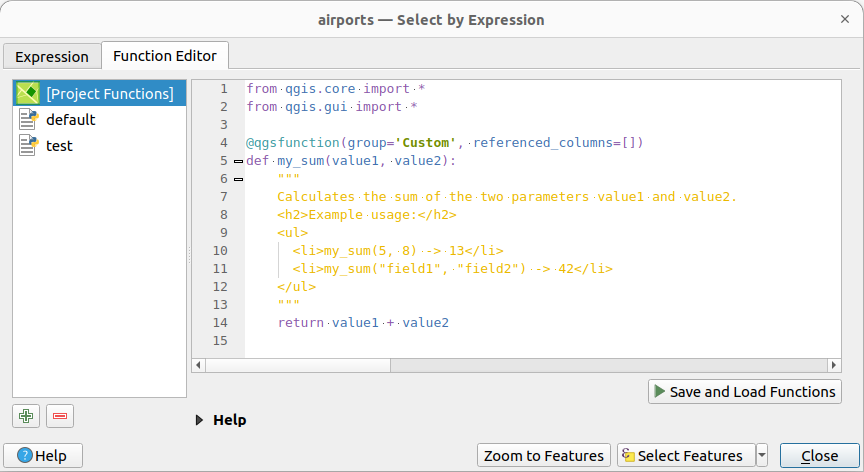The Function Editor tab

The function editor will create new Python files in .qgis2\python\expressions folder and will auto load all functions defined when starting QGIS. Be aware that new functions are only saved in the expressions folder and not in the project file. If you have a project that uses one of your custom functions you will need to also share the .py file in the expressions folder.

Aqui há um breve exemplo de como criar suas próprias funções:

```@qgsfunction(args="auto", group='Custom')
def myfunc(value1, value2, feature, parent):
pass
```

The short example creates a function ‘myfunc’ that will give you a function with two values. When using the args=’auto’ function argument the number of function arguments required will be calculated by the number of arguments the function has been defined with in Python (minus 2 - feature, and parent).

This function then can be used with the following expression:

```myfunc('test1', 'test2')
```

Your function will be implemented in the Custom functions group of the Expression tab after using the Run Script button.

Further information about creating Python code can be found in the Passo-a-passo para desenvolvedor PyQGIS.

The function editor is not only limited to working with the field calculator, it can be found whenever you work with expressions.